Pulse broadening discussed in the dispersion in single-mode fibers tutorial is based on an intuitive phenomenological approach. It provides a first-order estimate for pulses whose spectral width is dominated by the spectrum of the optical source rather than by the Fourier spectrum of the pulse. In general, the extent of the pulse broadening depends on the width and the shape of the input pulse. This tutorial discusses pulse broadening by using the wave equation derived previously.

#### 1. Basic Propagation Equation

The discussion of fiber modes showed that each frequency component of the optical field propagates in a single-mode fiber as a plane wave and can be written as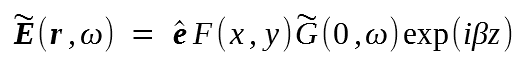where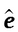is the polarization unit vector,is the initial amplitude, and β is the propagation constant. F(xy) is the field distribution of the fundamental fiber mode that can often be approximated by a Gaussian distribution. In general, F(xy) also depends on ω, but this dependence can be ignored for pulses whose spectral width Δω << ω0, a condition generally satisfied in practice. Here ωis the frequency at which the pulse spectrum is centered; it is referred to as the center frequency or the carrier frequency. Different spectral components propagate inside the fiber according to the simple relationThe amplitude in the time domain can be obtained by taking the inverse Fourier transform and is given byThe initial spectral amplitude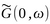is just the Fourier transform of the input amplitude G(0, t).

Pulse broadening results from the frequency dependence of β. For quasimonochromatic pulses with Δω << ω0, it is useful to expand β(ω) in a Taylor series around the carrier frequency ω0 and retain terms up to the third order, that iswhere Δωωω0 and βm = (dmβ/m)ω=ω0β1 = 1/vg, where vg is the group velocity. The GVD coefficient β2 is related to the dispersion parameter D, whereas β3 is related to the dispersion slope S.

We substitute some equations and introduce a slowly varying amplitude A(z, t) of the pulse envelope by the relation

G(z, t) = A(z, t) exp[(β0z - ω0t) ]

The amplitude A(z, t) is found to be given bywhere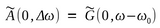is the Fourier transform of A(0, t).

The above equation can be written in the time domain as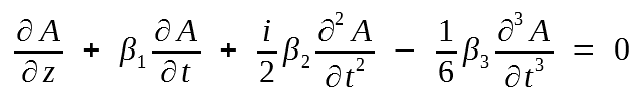by calculating ∂A/z and noting that Δω is replace by i(∂/∂t) in the time-domain description.

The above equation is the basic propagation equation that governs pulse evolution inside a dispersive fiber. In the absence of dispersion (β2β3 = 0),  pulse propagates without change in its shape such that A(z, t) = A(0, tβ1z).

By making the transformation to a reference frame moving with the pulse and introducing the new coordinates

t' = tβ1z   and   z' = z,

the above equation can be written as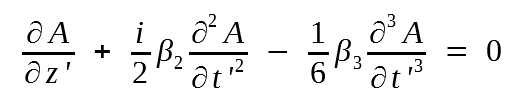For simplicity the prime over z' and t' is dropped in the following discussion.

#### 2. Gaussian Input Pulses

In this section we consider propagation of Gaussian input pulses in optical fibers by taking the initial amplitude aswhere A0 is the peak amplitude, and the parameter T0 represents the half-width at 1/e intensity point. It is related to the full width at half maximum (FWHM) by the relation

TFWHM = 2 (ln2)1/2 T0 ≈ 1.665T0

The parameter C governs the linear frequency chirp imposed on the pulse. A pulse is said to be chirped if its carrier frequency changes with time. The frequency change is related to the phase derivative and is given by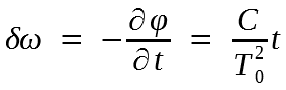where φ is the phase of complex amplitude A. The time-dependent frequency shift δω is called the chirp. Its inclusion is important, since semiconductor lasers generally emit pulses that are considerably chirped. The Fourier spectrum of a chirped pulse is broader than that of the unchirped pulse. This can be seen by taking the Fourier transform of the initial amplitude A(0, t) so that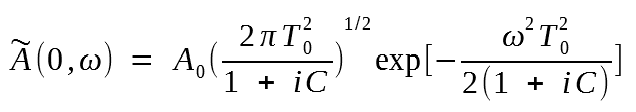The spectral half-width (at 1/e intensity point) is given by

Δω0 = (1 + C2)1/2/T0

In the absence of frequency chirp (C = 0), the spectral width satisfies the relation Δω0T0 = 1. Such a pulse has the narrowest spectrum and is called transform-limited. The spectral width is enhanced by a factor of (1 + C2)1/2 in the presence of linear chirp as seen in the last equation.

The pulse-propagation equation can be easily solved in the Fourier domain. Its solution is given bywhereis given from above for the Gaussian input pulse. Let us first consider the case in which the carrier wavelength is far away from the zero-dispersion wavelength so that the contribution of the β3 term is negligible. The integration from the last equation can be performed analytically, and the result is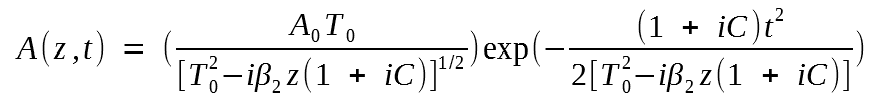This equation shows that a Gaussian pulse remains Gaussian on propagation. The pulse width changes with z aswhere T1 is the half-width at 1/e intensity point defined similar to T0.

The following figure shows the broadening factor T1/T0 as a function of the propagation distance z/LD, where LD = T02/|β2| is called the dispersion length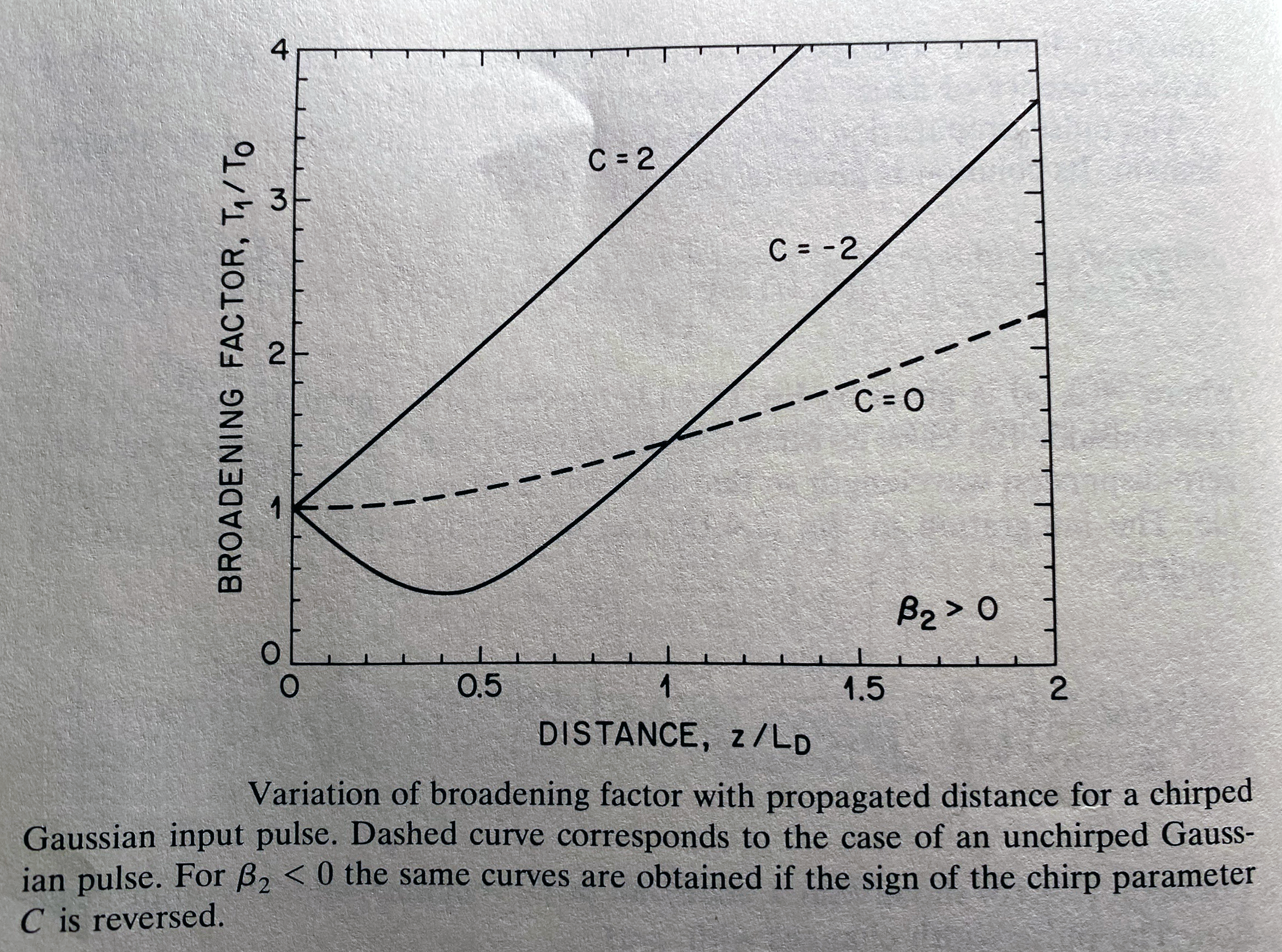An unchirped pulse (C = 0) broadens as [1 + (z/LD)2]1/2 and its width increases by a factor of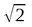at z = LD. The chirped pulse, on the other hand, may broaden or compress depending on whether β2 and C have the same or opposite signs. For β2C > 0 the chirped Gaussian pulse broadens monotonically at a rate faster than that the unchirped pulse. For β2C < 0 the pulse width initially decreases and becomes minimum at a distance

zmin = [C/(1 + C2)]LD

The minimum value depends on the chirp parameter as

T1min = T0/(1+C2)1/2

As we shall see later, initial pulse narrowing of suitably chirped pules can be used to advantage in the design of optical communication systems.

The T1/T0 equation above can be generalized to include higher-order dispersion governed by β3. The integral can still be performed in a closed form in terms of an Airy function.  The Gaussian input pulse does not remain Gaussian on propagation and develops a long tail with an oscillatory structure. Such pulses cannot be properly characterized by their FWHM or 1/e intensity width. A proper measure of the pulse width is the root-mean-square (rms) width of the pulse defined by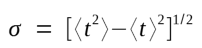where angle brackets denote averaging with respect to the intensity profile, that is,The broadening factor defined as σ/σ0, where σ0 is the rms width of the input Gaussian pulse (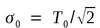), is given bywhere L is the fiber length.

The foregoing discussion assumes that the optical source used to produce the input pulses is nearly monochromatic such that its spectral width satisfies ΔωL << Δω0 (under continuous-wave [cw] operation),  where Δω0 is given by previous equation. This condition is often not satisfied in practice. To account for the source spectral width, one must treat the optical field as a stochastic process and consider the coherent properties of the source through the mutual coherence function. The average in σ then includes an average over the statistical nature of the source. For a Gaussian source spectrum with a rms spectral width σω, it is possible to obtain the broadening factor in an analytic form with the resultwhere V is defined by V = 2σωσ.  This equation provides an expression for dispersion-induced pulse broadening of Gaussian input pulses under quite general conditions. We use it in the next section to obtain the limiting bit rate of optical communication systems employing single-mode fibers as a communication channel.

#### 3. Limitations on the Bit Rate

The limitation imposed on the bit rate by fiber dispersion can be quite different depending on the source spectral width. It is instructive to consider the following two cases separately.

Case (a): Large Source Spectral Width

This case corresponds to V >> 1 in the above equation. Consider first the case of a lightwave system operating away from the zero-dispersion wavelength so that the β3 term can be neglected. If we further neglect the frequency chirp (C = 0), the broadening factor in the above equation can be approximated by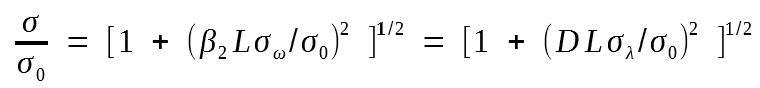where σλ is the rms source spectral width in wavelength units. The output width is given by

σ = (σ02 + σD2)1/2

where σD = |D|λ is a measure of dispersion-induced broadening.

We can relate σ to the bit rate by using the criterion that the broadened pulse should remain inside the allocated bit slot TB = 1/B, where B is the bit rate. A commonly used criterion is σ <= TB/4; for Gaussian pulses at least 95% of the pulse energy then remains within the bit slot. The limiting bit rate is given by 4Bσ <= 1.  For short input pulses σ ≈ σD = |D|Lσλ , and the condition becomes

BL|D|Lσλ <= 1/4

For a lightwave system operating exactly at the zero-dispersion wavelength, β2 = 0. If we neglect the frequency chirp by setting C = 0 and take V >> 1, we can then obtain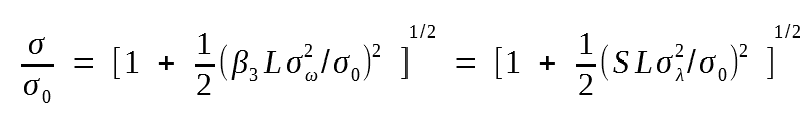where we have related β3 to the dispersion slope S. The output pulse width is thus given by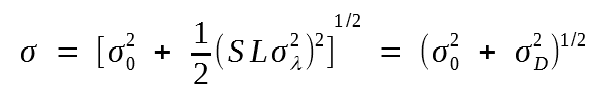whereAs before, we can relate σ to the limiting bit rate by the condition 4Bσ <= 1. When σ0 << σD, the limitation on the bit rate is given by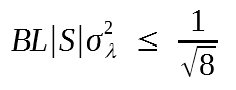Case (b): Small Source Spectral Width

This case corresponds to V << 1, since the source spectral width σω is assumed to be much smaller than 1/σ0. If we neglect the β3 term and set C = 0, we can obtainA comparison reveals a major difference between the two cases. For a narrow source spectrum, dispersion-induced broadening depends on the initial width σ0, whereas it is independent of σ0 when the spectral width of the optical source dominates. In fact, σ can be minimized by choosing an optimum value of σ0. The minimum value of σ is found to occur for σ0 = σD = (|β2|L/2)1/2 and is given by σ = (|β2|L)1/2. The limiting bit rate can be obtained by using 4 <= 1 and leads to the condition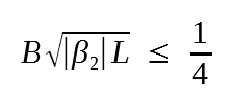The main difference from the large source spectral width is that B scales as L-1/2 rather than L-1.

The following figure compares the decrease in the bit rate with increasing L by choosing D = 16 ps/(km-nm) for σλ = 0, 1, and 5 nm. The above equation was used for the case σλ = 0.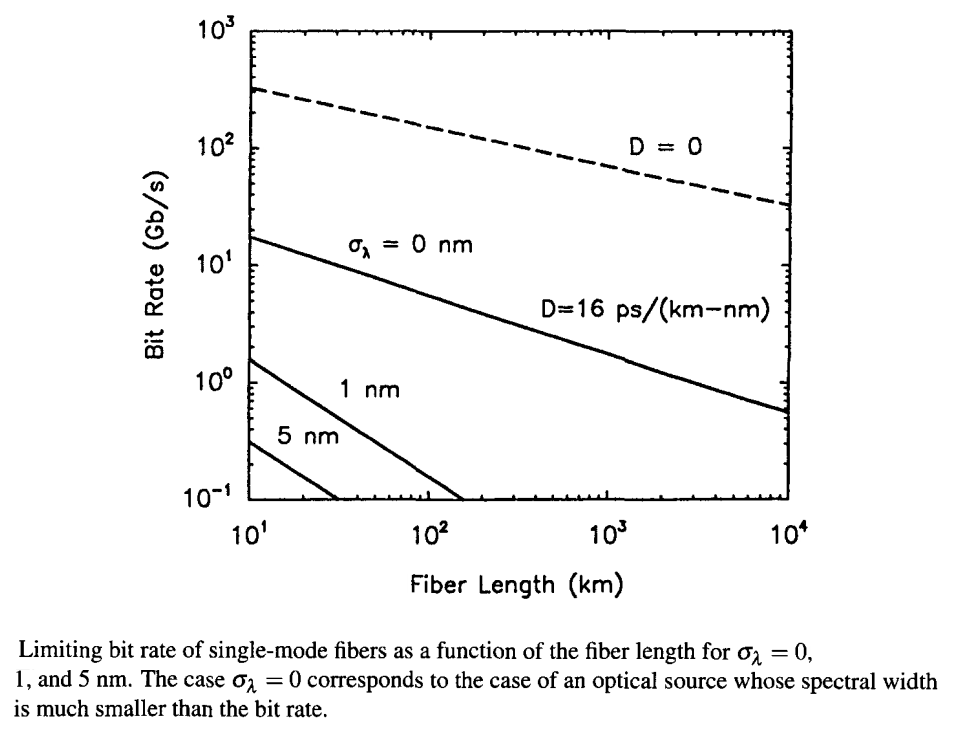For a lightwave system operating exactly at the zero-dispersion wavelength,  β2 = 0. By using V << 1 and C << 1, the pulse width is then given by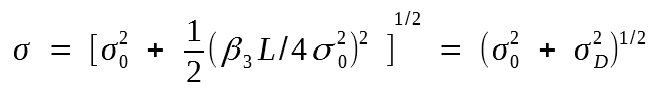Here σ can be minimized by optimizing the input pulse width σ0. The minimum value of σ is found to occur for σ0 = (|β3|L/4)1/3 and is given by

σ = (3/2)1/2(|β3|L/4)1/3

The limiting bit rate is obtained by using the condition 4 <= 1 and is given by

B(|β3|L)1/3 <= 0.324

The dispersive effects are most forgiving in this case. For a typical value β3 = 0.1 ps3/km, the bit rate can be as large as 150 Gb/s for L = 100 km. It decreases to only about 70 Gb/s even when L increases by a factor of 10 because of the L-1/3 dependence of the bit rate on the fiber length. The dashed line in the figure above shows this dependence with β3 = 0.1 ps3/km. Clearly, the performance of lightwave systems can be considerably improved by operating near the zero-dispersion wavelength of the fiber and by using optical sources with a relatively narrow spectral width.

The input pulse in both cases (a) and (b) has been assumed to be an unchirped Gaussian pulse. In practice, optical pulses emitted from semiconductor lasers are non-Gaussian and exhibit considerable chirp. A super-Gaussian model has been used to study the bit-rate limitation imposed by fiber dispersion for such input pulses. Equation is then replaced bywhere the parameter m controls the pulse shape. Chirped Gaussian pulses correspond to m = 1. For larger values of m the pulse becomes nearly rectangular with sharper leading and trailing edges. The output pulse shape can be obtained by solving numerically. The limiting bit rate-distance product BL is found by requiring that the rms pulse width does not increase above a tolerable broadening factor.

The following figure shows the BL product as a function of the chirp parameter C for Gaussian (= 1) and super-Gaussian (m = 3) input pulses. In both cases the fiber length L at which the pulse broadens by 20% was obtained for T0 = 125 ps and β2 = -20 ps2/km.As expected, the BL product is smaller for super-Gaussian pulses because such pulses broaden more rapidly than Gaussian pulses. The BL product is dramatically reduced for negative values of the chirp parameter C. This is due to enhanced broadening occurring when β2C is positive. Unfortunately, C is generally negative for directly modulated semiconductor lasers with a typical value of -6 at 1.55 μm. Since BL < 100 (GB/s) under such conditions, fiber dispersion limits the bit rate to about 2 Gb/s for L = 50 km. This problem can be overcome by using dispersion-shifted fibers.

#### 4. Fiber Bandwidth

The concept of fiber bandwidth originates from the general theory of time-invariant linear systems. If the optical fiber can be treated as a linear system, its input and output powers should be related by a general relation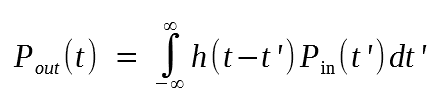For an impulse Pin(t) = δ(t), where δ(t) is the delta function, and Pout = h(t). For this reason, h(t) is called the impulse response of the linear system. Its Fourier transform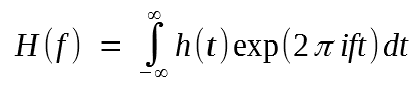provides the frequency response and is called the transfer function. In general, |H(f)| falls off with increasing f, indicating that the high-frequency components of the input signal are attenuated by the fiber. In effect, the optical fiber acts as a bandpass filter. The fiber bandwidth f3 dB corresponds to the frequency f = f3 dB at which |H(f)| is reduced by a factor of 2 or by 3 dB, that is

|H(f3 dB)H(0)| = 1/2

Note that  f3 dB is the optical bandwidth of the fiber as the optical power drops by 3 dB at this frequency compared with the zero-frequency response. In the theory of electrical communications, the bandwidth of a linear system is generally defined as the frequency at which electrical power drops by 3 dB. Since the optical power converts to electric current, the 3-dB electrical bandwidth is obtained by replacing the factor of 1/2 in the above equation by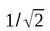.

Optical fibers cannot generally be treated as linear systems and the first equation does not hold for them. However, they can be approximated by a linear system if the source spectral width ΔωL is much larger than the signal spectral width Δω0. One can then consider propagation of different spectral components independently and add the power carried by them linearly to obtain the output power. For a Gaussian spectrum, the transfer function H(f) is found to be given by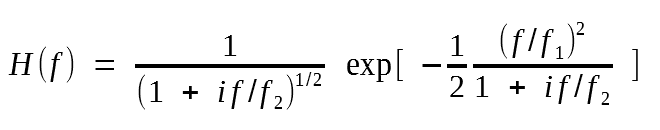where

f1 = (2πβ2ω)-1 = (2π|D|λ)-1

f2 = (2πβ3ω2)-1 = [2π(S + 2D/λ)λ2]-1

and we used previous equations to introduce the dispersion parameters D and S.

For lightwave systems operating far away from the zero-dispersion wavelength (f1 << f2), the transfer function is approximately Gaussian. By using two previous equations with f << f2, the fiber bandwidth is given by

f3 dB = (2 ln2)1/2f1 ≈ 0.188(|D|λ)-1

If we use σD = |D|λ, we obtain the relation f3 dBσD ≈ 0.188 between the fiber bandwidth and dispersion-induced pulse broadening. We can also get a relation between the bandwidth and the bit rate B which is B <= 1.33f3 dB and shows that the fiber bandwidth is an approximate measure of the maximum possible bit rate of dispersion-limited lightwave systems. In fact, the 2nd last figure can be used to estimate f3 dB and its variation with the fiber length under different operating conditions.

For lightwave systems operating at the zero-dispersion wavelength the transfer function is obtained from the H(f) equation above by setting D = 0. We then get the following expression for the fiber bandwidth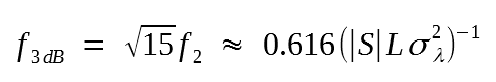The limiting bit rate can be related to f3 dB and is given by B <= 0.574f3 dB. Again, the fiber bandwidth provides a measure of the dispersion-limited bit rate. As a numerical estimate, consider a 1.55 μm lightwave system employing dispersion-shifted fibers and multimode semiconductor lasers. By using S = 0.05 ps/(km-nm2) and σλ = 1 nm as typical values, f3 dBL ≈ 32 THz-km. By contrast, the bandwidth-distance product is reduced to 100 GHz-km for standard fibers with D = 18 ps/(km-nm).